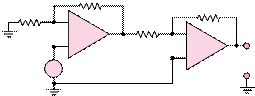### Create an Account

Home / Questions / Find an expression for the output voltage in the circuit of Figure P844 RF 1 circui...

# Find an expression for the output voltage in the circuit of Figure P844 RF 1 circuit of Figure P832 Assume that R3 R4 and R1 R2 How closely can you approximate the desired gain

Find an expression for the output voltage in the circuit of Figure P8.44.

R1

circuit of Figure P8.32. Assume that R3 = R4 and

R1  = R2.

How closely can you approximate the desired

gain? Compute the error in the gain, assuming that the 1 percent tolerance resistors have the nominal value.

RS1

+

 _

vs    +

RS2

R2+

+                  vo

8.51 Repeat Problem 8.50, but use the ±1 percent tolerance range to compute the possible range of gains for this amplifier. You may assume that R3  = R4 and R1  = R2.

Jun 18 2020 View more View LessSubscribe To Get Solution